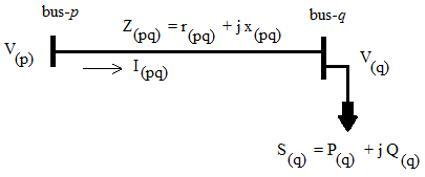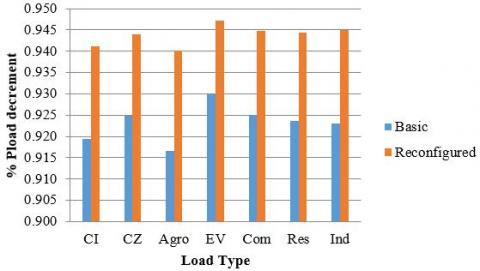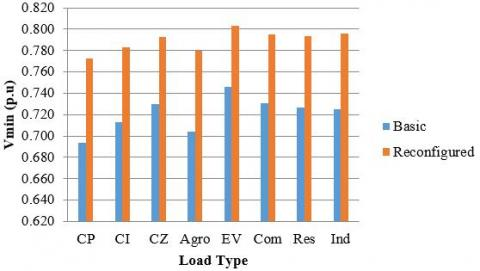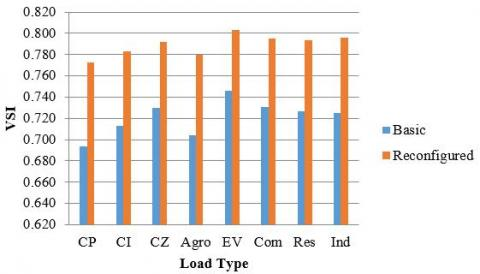# Static Voltage Stability of Reconfigurable Radial Distribution System Considering Voltage Dependent Load Models

Static Voltage Stability of Reconfigurable Radial Distribution System Considering Voltage Dependent Load Models
Dept. of Electrical and Electronics Engineering, School of Engineering and Technology, CHRIST (Deemed to be University), Bangalore 560074, Karnataka, India

Dept. of Electrical and Electronics Engineering, Dhanekula Institute of Engineering & Technology, Vijayawada 521139, Andhra Pradesh, India

Corresponding Author Email:
Page:
450-458
|
DOI:
https://doi.org/10.18280/mmep.070316
9 June 2020
|
Accepted:
23 August 2020
|
Published:
30 September 2020
| Citation

OPEN ACCESS

Abstract:

This paper presents the static voltage stability analysis of RDS. Initially the performance of RDS is evaluated using backward/forward load flow considering voltage-dependent load modeling. Later, the load flow solution is used for determining the static voltage stability of the system. The analysis is performed for different type of loads such as constant power, constant current, constant impedance, residential, industrial, commercial, agricultural and electric vehicle loads. The simulations are performed for standard and optimal reconfigured topology of standard IEEE 33-bus test system. The comparative study reveals the importance of load type and topology while assessing the static stability analysis of radial distribution systems.

Keywords:

1. Introduction

In similar, different indices for assessing voltage stability of the distribution systems can be found in literature . From the aforementioned literature, it is observed that the majority of works have been used mainly constant power (CP) load model in their methodology. A limited works have also analyzed using constant current (CI) and constant impedance (CZ) load models. But none of them have focused on the residential, industrial and commercial type of load models. In addition, the literature is also not focused the agriculture and electric vehicle (EV) loads. According to separate feeder concept for agriculture sector and increasing high EV load penetrations, assessment of voltage stability is essential. This paper made an attempt to focus on voltage stability analysis considering the above mentioned distinguished type of load models. In the literature, it is also proved that the network topology can also influence the system performance and voltage stability significantly .

In light of the above reviewed works, the static voltage stability analysis of radial distribution system is highly dependent on its configuration, type of load and loading conditions. In this paper, the impact of different types of loads is analyzed on IEEE 33-bus system considering its basic and optimal configurations. The rest of the paper is arranged as follows: Section 2 presents the mathematical modeling involved in static voltage stability analysis of RDA. Section 3 explains the voltage dependent modeling for different types of loads. Section 4 presents the simulation results of IEEE 33-bus system for basic and optimized configurations. In Section 5, the major research findings and observations are presented.

2. Voltage Stability Assessment

The stability index proposed by Chakravorty and Das  is used for assessing the static voltage stability analysis of the system. A typical two-bus test system interconnected by a branch having impedance of Z(pq) is shown in Figure 1.

## 1.jpgFigure 1. Typical two-bus RDS network

The complex power connected at bus-q is S(q)=P(q)+jQ(q). The current flow through branch, I(pq) is equivalnet to the current drawn by the load at bus-q, I(q) and is given by,

${{I}_{\left( pq \right)}}={{I}_{\left( q \right)}}={{\left( {{{S}_{\left( q \right)}}}/{{{V}_{\left( q \right)}}}\; \right)}^{*}}$    (1)

$=\frac{{{P}_{\left( q \right)}}-j{{Q}_{\left( q \right)}}}{\left| {{V}_{\left( q \right)}} \right|\angle -{{\delta }_{\left( q \right)}}}=\frac{\left| {{V}_{\left( p \right)}} \right|\angle {{\delta }_{\left( p \right)}}-\left| {{V}_{\left( q \right)}} \right|\angle {{\delta }_{\left( q \right)}}}{{{r}_{\left( pq \right)}}+j{{x}_{\left( pq \right)}}}$   (2)

By simplification, Eq. (2) can be written as,

$\left\{ \left| {{V}_{\left( p \right)}} \right|\angle {{\delta }_{\left( p \right)}}-\left| {{V}_{\left( q \right)}} \right|\angle {{\delta }_{\left( q \right)}} \right\}\left( \left| {{V}_{\left( q \right)}} \right|\angle -{{\delta }_{\left( q \right)}} \right)=$   $\left( {{P}_{\left( q \right)}}-j{{Q}_{\left( q \right)}} \right)\left( {{r}_{\left( pq \right)}}+j{{x}_{\left( pq \right)}} \right)$  (3)

$\left\{ \left| {{V}_{\left( p \right)}} \right|\left| {{V}_{\left( q \right)}} \right|\angle \left( {{\delta }_{\left( p \right)}}-{{\delta }_{\left( q \right)}} \right) \right\}-{{\left| {{V}_{\left( q \right)}} \right|}^{2}}=$       $\left( {{P}_{\left( q \right)}}-j{{Q}_{\left( q \right)}} \right)\left( {{r}_{\left( pq \right)}}+j{{x}_{\left( pq \right)}} \right)$        (4)

By separating real and imaginary parts, we have,

$\left| {{V}_{\left( p \right)}} \right|\left| {{V}_{\left( q \right)}} \right|\cos \left( {{\delta }_{\left( pq \right)}} \right)={{P}_{\left( q \right)}}{{r}_{\left( pq \right)}}+{{Q}_{\left( q \right)}}{{x}_{\left( pq \right)}}+{{\left| {{V}_{\left( q \right)}} \right|}^{2}}$        (5)

$\left| {{V}_{\left( p \right)}} \right|\left| {{V}_{\left( q \right)}} \right|\sin \left( {{\delta }_{\left( pq \right)}} \right)={{P}_{\left( q \right)}}{{x}_{\left( pq \right)}}-{{Q}_{\left( q \right)}}{{r}_{\left( pq \right)}}$        (6)

Squaring and adding Eq. (5) and Eq. (6) and then simplifying, we have

${{\left| {{V}_{\left( q \right)}} \right|}^{4}}-{{k}_{1}}{{\left| {{V}_{\left( q \right)}} \right|}^{2}}+{{k}_{2}}=0$      (7)

where,

${{k}_{1}}={{\left| {{V}_{\left( p \right)}} \right|}^{2}}-2{{P}_{\left( q \right)}}{{r}_{\left( pq \right)}}-2{{Q}_{\left( q \right)}}{{x}_{\left( pq \right)}}$    (8)

${{k}_{2}}=\left( P_{\left( q \right)}^{2}+Q_{\left( q \right)}^{2} \right)\left( r_{\left( pq \right)}^{2}+x_{\left( pq \right)}^{2} \right)$       (9)

From the feasible solution for Eq. (7), the voltage magnitude of bus-q and its phase angles are given by:

$\left| {{V}_{(q)}} \right|={{\left[ \frac{1}{2}{{k}_{1}}+{{\left( k_{1}^{2}-4{{k}_{2}} \right)}^{\frac{1}{2}}} \right]}^{\frac{1}{2}}}$      (10)

${{\delta }_{\left( q \right)}}={{\delta }_{\left( p \right)}}-{{\tan }^{-1}}\left[ \frac{\left( {{P}_{\left( q \right)}}{{x}_{\left( pq \right)}}-{{Q}_{\left( q \right)}}{{r}_{\left( pq \right)}} \right)}{{{\left| {{V}_{\left( q \right)}} \right|}^{2}}+{{P}_{\left( q \right)}}{{r}_{\left( pq \right)}}+{{Q}_{\left( q \right)}}{{x}_{\left( pq \right)}}} \right]$       (11)

Using voltage profile, the real and reactive powers of a branch and consequently total system losses can determine using the following:

${{P}_{loss\left( pq \right)}}={{r}_{\left( pq \right)}}\left( \frac{P_{\left( q \right)}^{2}+Q_{\left( q \right)}^{2}}{{{\left| {{V}_{\left( q \right)}} \right|}^{2}}} \right)$        (12)

${{Q}_{loss\left( pq \right)}}={{x}_{\left( pq \right)}}\left( \frac{P_{\left( q \right)}^{2}+Q_{\left( q \right)}^{2}}{{{\left| {{V}_{\left( q \right)}} \right|}^{2}}} \right)$        (13)

${{P}_{T,loss}}=\sum\limits_{pq=1}^{nbr}{{{P}_{loss\left( pq \right)}}}$       (14)

${{Q}_{T,loss}}=\sum\limits_{pq=1}^{nbr}{{{Q}_{loss\left( pq \right)}}}$       (15)

where, |V(p)| and |V(q)| are the voltage magnitudes at bus-p and bus-q respectively; δ(p) and δ(q) are the load angles of bus-p and bus-q respectively; r(pq) and x(pq) are the resistance and reactance of the branch impedance respectively; P(q) and Q(q) are the real and reactive power loads at bus-q respectively. Ploss(pq) and Qloss(pq) are the real and reactive power losses in branch p-q respectively; PT,loss and QT,loss are the real and reactive power losses of the total distribution system respectively; nbr is number of branches in the network.

Initially, Ploss(pq) and Qloss(pq) for all branches are set to zero. By knowing bus-p voltage magnitude and its load angle (|V(p)|=1.00p.u. & δ(p)=0), the initial effective powers P(q) and Q(q) are determined by summing all the loads beyond bus-q plus local load of bus-q. Later, the real and reactive power losses of branch pq are determined using Eq. (12) and Eq. (13). By proceeding for all the branches, the total power loss of the system can be determined using Eq. (14) and Eq. (15). This stage will complete one iteration. By updating the bus-injections P(q)=P(q)+Ploss(pq) and Q(q)=Q(q)+Qloss(pq), the procedure continues until satisfies the convergence criterion, error, ε is defined as $\max \left( \left| V_{\left( n \right)}^{itr+1} \right|-\left| V_{\left( n \right)}^{itr} \right| \right)\le {{10}^{-5}}$ (i.e., the maximum of change in voltage magnitudes between two consecutive iterations of all buses).

The feasible solution for Eq. (9) can exist only by satisfying the following condition,

$k_{1}^{2}-4{{k}_{2}}\ge 0$       (16)

After substituting k1 and k2 as defined in Eq. (8) & Eq. (9), Eq. (16) can be written as,

${{\left| {{V}_{(q)}} \right|}^{4}}-4{{\left( {{x}_{\left( pq \right)}}{{P}_{eff\left( q \right)}}-{{r}_{\left( pq \right)}}{{Q}_{eff\left( q \right)}} \right)}^{2}}-$

$4\left( {{r}_{\left( pq \right)}}{{P}_{eff\left( q \right)}}+{{x}_{\left( pq \right)}}{{Q}_{eff\left( q \right)}} \right){{\left| {{V}_{(p)}} \right|}^{2}}_{2}\ge 0$      (17)

where, Peff(q) and Qeff(q) are the effective real and reactive loadings at bus-q, which include connected load and losses.

Therefore, voltage stability index (VSI) for bus-q is defined by,

$VS{{I}_{\left( q \right)}}={{\left| {{V}_{(q)}} \right|}^{4}}-4{{\left( {{x}_{\left( pq \right)}}{{P}_{eff\left( q \right)}}-{{r}_{\left( pq \right)}}{{Q}_{eff\left( q \right)}} \right)}^{2}}$

$4\left( {{r}_{\left( pq \right)}}{{P}_{eff\left( q \right)}}+{{x}_{\left( pq \right)}}{{Q}_{eff\left( q \right)}} \right){{\left| {{V}_{(p)}} \right|}^{2}}_{2}\ge 0$     (18)

For the stable operation, VSI(q)≥0, for q = 1, 2, …, nbus. The lowest VSI(q) among all buses is said to be the overall system stability condition.

In conventional load studies, the loads are presumed constant irrespective of their associated bus voltage magnitude. In contrast, the voltage profile of the distribution system is always varying due to distinct change changes in the load profile from low to high. Also, the distribution system voltage profile subjected to change w.r.t. type of load. In general, the distribution systems serve different type of loads and their characteristics and profile are not same. Due to these, the power consumption by different types of loads may not be constant as mentioned on their name plate, but continuously vary w.r.t. the changes in voltage magnitude and frequency of the associated bus.  In order to accommodate this fact, the power ratings of different loads have been modeled using voltage-dependent load modeling. According to this, the real and reactive power demands of a load are given by:

${{P}_{d,i}}=P_{d,i}^{\left( 0 \right)}\times {{\left( {\left| {{V}_{i}} \right|}/{\left| V_{i}^{\left( 0 \right)} \right|}\; \right)}^{\alpha }}$     (19)

${{Q}_{d,i}}=Q_{d,i}^{\left( 0 \right)}\times {{\left( {\left| {{V}_{i}} \right|}/{\left| V_{i}^{\left( 0 \right)} \right|}\; \right)}^{\beta }}$    (20)

where, $P_{d,i}^{\left( 0 \right)}$ and $Q_{d,i}^{\left( 0 \right)}$  are the active and reactive power loads at bus-i at nominal voltage respectively; Pd,i and Qd,i are active and reactive power loads at actual voltage respectively; $\left| {{V}_{i}} \right|$and $\left| V_{i}^{\left( 0 \right)} \right|$  are the actual voltage magnitude and nominal voltage magnitudes respectively; α and β are the exponents of active and reactive power loads respectively. The exponents for Constant power (CP), Constant current (CI), Constant impedance (CZ), Agricultural load (AL) (i.e., pumps, fans other motors) can be found . The exponents for Electric vehicle (EV) load are given by Satyanarayana et al. , Kongjeen and Bhumkittipich . The exponents for residential (RL), industrial (IL) and commercial (CL) loads are given by Abdi and Afshar .

4. Results and Discussion

The proposed methodology for evaluating the static voltage stability of RDSs is applied on the standard 33-bus balanced test system. The voltage stability is computed for the basic topology and optimal reconfigured topologies for different load models. The optimal reconfigured topology is taken from the existing literature . The single line diagram of test system for basic topology is given in Figure 2. The test system has 32-branches (black color lines) and 5-tie-lines (dotted lines) . For the analysis purpose, constant power, constant current, constant impedance, residential, industrial, commercial, agricultural, electric vehicle loads are considered in this work. The exponentials of active and reactive powers for different loads are given in Table 1.

Generally, the optimal network reconfiguration subjected to operational constraints, radiality constraints and aimed to optimize an objective function like loss minimization, voltage stability enhancement, loadability enhancement. In this paper, the optimal configuration of IEEE 33-bus is directly taken as obtained in many literature paper .

The optimal reconfiguration of the test system is arrived by closing the tie-lines 33 (8-21), 34 (9-15), 35 (12-22) & 36 (18-33) and by opening the branches 7 (7-8), 9 (9-10), 14 (14-15) & 32 (32-33) and tie-line 37 (25-29 respectively. The optimal reconfiguration with branch sequence for BW/FW load flow study is given in Figure 3.

The performance is measured in terms of active and reactive power losses, voltage profile, and voltage stability index. The results for different types of loads for basic topology and optimized topology are given in Table 2 and Table 3 respectively. In Table 2 and Table 3, column 1 represents the type of load, column 2 and 3 represent the total real and reactive power loads of the network respectively, column 4 and 5 represent the total real and reactive power distribution losses respectively; column 6 represnts the voltage stability of the system and column 7 indicates the lowest voltage magnitude in the entire system with associated bus details.

Table 1. Exponentials of active and reactive powers for different loads

 Load α β Load Α β CP 0 0 IL 0.18 6 CI 1 1 CL 1.51 3.4 CZ 2 2 AL 0.08 1.60 RL 0.92 4.04 EV 2.59 4.06

At nominal voltage profile, the test system has real power load of 3715 kW and reactive load of 2300 kVAr respectively. For the basic topology, the load flow resulted for total real and reactive power losses around 202.67 kW and 135.14 kVAr respectively. The average voltage deviation index (AVDI) and voltage stability index (VSI) are 0.0035 and 0.694 respectively. The AVDI is calculated w.r.t. reference/sub-station bus voltage i.e., 1.0 p.u. Also, the system is observed with lowest voltage magnitude of 0.9131 p.u. at bus-18. The load flow is performed for different types of loads as mentioned in section 2. The effective new loading with voltage-dependent load modeling and correspondingly system performance are given in Table 2.

The load flow study is repeated for all types of loads considering optimal topology as given in Figure 3. At nominal voltage profile, the test system loading conditions are remaining same but the performance is improved significantly. The active power losses are decreased to 139.53 kW from 202.67 kW, reactive power losses are decreased to 102.29 kVAr from 135.14 kVAr, the AVDI is decreased to 0.0015 from 0.0035, VSI is improved to 0.7722 from 0.694 and the lowest voltage magnitude at bus-18 is raised to 0.9378 p.u. from 0.9131 p.u. Hence, it can be said that the optimal topology has resulted for improved performance in all the aspects. The analysis is carried for different types of loads considering optimal topology and is given in Table 3.

## 2.jpgFigure 2. The basic topology of IEEE 33-bus test system 

## 3.jpgFigure 3. The optimal reconfigured IEEE 33-bus test system 

Table 2. The performance analysis and voltage stability analysis under standard topology

 Load Pload (kW) Qload (kVAr) Ploss (kW) Qloss (kVAr) AVDI VSI Vmin (p.u.) CP 3715.00 2300.00 202.67 135.14 0.0035 0.6940 0.9131 CI 3543.25 2181.01 176.62 117.54 0.0031 0.7132 0.9194 CZ 3382.25 2069.91 165.96 109.69 0.0027 0.7300 0.9248 AL 3700.44 2108.14 186.82 124.37 0.0033 0.7044 0.9165 EV 3291.71 1862.49 159.49 95.73 0.0023 0.7460 0.9299 CL 3459.99 1925.93 170.45 99.87 0.0027 0.7308 0.9250 RL 3556.79 1864.37 176.47 95.65 0.0027 0.7264 0.9236 IL 3683.33 1690.95 184.08 83.95 0.0027 0.7249 0.9231

Table 3. The performance analysis and voltage stability analysis under optimized topology
 Load Pload (kW) Qload (kVAr) Ploss (kW) Qloss (kVAr) AVDI VSI Vmin (p.u.) CP 3715.00 2300.00 139.53 102.29 0.0015 0.7722 0.9378 CI 3589.73 2212.42 127.48 93.41 0.0013 0.7828 0.9411 CZ 3470.13 2129.16 122.08 89.24 0.0012 0.7923 0.9439 AL 3704.61 2159.56 131.67 96.67 0.0014 0.7797 0.9401 EV 3401.65 1968.77 118.81 81.69 0.0011 0.8031 0.9472 CL 3528.28 2018.53 124.23 84.16 0.0012 0.7948 0.9447 RL 3599.86 1970.26 127.11 82.01 0.0012 0.7937 0.9443 IL 3692.13 1830.80 130.66 75.69 0.0012 0.7958 0.9450

## 4.jpgFigure 4. % Change in Pload for different types of loads under basic and optimized topology

## 5.jpgFigure 5. % Change in Qload for different types of loads under basic and optimized topology

## 6.jpgFigure 6. % Ploss decrement for different types of loads under basic and optimized topology

## 7.jpgFigure 7. % Qloss decrement for different types of loads under basic and optimized topology

## 8.jpgFigure 8. Vmin for different types of loads under basic (at bus-18) and optimized topology (at bus-32)

## 9.jpgFigure 9. VSI under different types of loads for basic and optimized topology

5. Conclusion

References

 Alhelou, H.H., Hamedani-Golshan, M.E., Njenda, T.C., Siano, P. (2019) A survey on power system blackout and cascading events: research motivations and challenges, Energies, 12(4): 682. https://doi.org/10.3390/en12040682

 Nagendra, P., Datta, T., Halder, S., Paul, S. (2010). Power system voltage stability assessment using network equivalents – a review. Journal of Applied Sciences, 10(18): 2147-2153.

 Modarresi, J., Gholipourn, E., Khodabakhshian, A. (2016). A comprehensive review of the voltage stability indices. Renewable and Sustainable Energy Reviews, 63: 1-12. https://doi.org/10.1016/j.rser.2016.05.010

 Chakravorty, M., Das, D. (2001). Voltage stability analysis of radial distribution networks. International Journal of Electrical Power & Energy Systems, 23(2): 129-135. https://doi.org/10.1016/S0142-0615(00)00040-5

 Das, D., Kothari, D.P., Kalam, A. (1995). A simple and efficient method for load flow solution of radial distribution networks. International Journal of Electrical Power and Energy Systems, 17(5): 335-346. https://doi.org/10.1016/0142-0615(95)00050-0

 Eminoglu, U., Hocaoglu, M.H. (2007). A voltage stability index for radial distribution networks. 42nd International Universities Power Engineering Conference, Brighton, UK. https://doi.org/10.1109/UPEC.2007.4468982

 Eminoglu, U., Hocaoglu, M.H. (2009). A network topology-based voltage stability index for radial distribution networks. International Journal of Power and Energy Systems, 29(2): 131-143. https://doi.org/10.2316/Journal.203.2009.2.203-4280

 Mahmoud, G.A. (2012). Voltage stability analysis of radial distribution networks using catastrophe theory. IET Gener. Transm. Distrib., 6(7): 612-618. https://doi.org/10.1049/iet-gtd.2011.0530

 Ranjan, R., Das, D. (2003). Voltage stability analysis of radial distribution networks. Electric Power Components and Systems, 31(5): 501-511. https://doi.org/10.1080/15325000390127011

 Beigvand, S.D., Abdi, H., Singh, S.N. (2017). Voltage stability analysis in radial smart distribution grids. IET Gener. Transm. Distrib., 11(15): 3722-3730. https://doi.org/10.1049/iet-gtd.2016.1753

 Chakraborty, K., Deb, G., Deb, S. (2016). Voltage stability assessment in radial distribution system by line stability indicator (LSI) and its improvement using SVC. 2016 IEEE 1st International Conference on Power Electronics, Intelligent Control and Energy Systems (ICPEICES), Delhi, India. https://doi.org/10.1109/ICPEICES.2016.7853132

 Pitchaimuthu, R., Selvan, M.P., Kumaresan, N. (2013). Enhancement of voltage stability margin in radial distribution system with squirrel cage induction generator based distributed generators. IET Generation, Transmission & Distribution, 7(8): 898-906. https://doi.org/10.1049/iet-gtd.2012.0579

 Kayal, P., Chanda, C.K., Dutta, T. (2012). Efficient distribution system realization using equivalent voltage stability indicator. IACSIT International Journal of Engineering and Technology, 4(3): 270-274.

 Kumar, P., Singh, S. (2014). Comprehensive stability analysis of radial distribution system with load growth. 2014 IEEE 6th India International Conference on Power Electronics (IICPE), Kurukshetra, India pp. 1-6. https://doi.org/10.1109/IICPE.2014.7115733

 Jasmon, G.B., Lee, L.H.C.C. (1991). Distribution network reduction for voltage stability analysis and load flow calculations. International Journal of Electrical Power & Energy Systems, 13(1): 9-13. https://doi.org/10.1016/0142-0615(91)90011-J

 Moghavvemi, M., Faruque, M.O. (2001). Technique for assessment of voltage stability in ill-conditioned radial distribution network. IEEE Power Engineering Review, 21(1): 58-60. https://doi.org/10.1109/39.893345

 Hamada, M.M., Wahab, M.A.A., Hemdan, N.G.A. (2010). Simple and efficient method for steady-state voltage stability assessment of radial distribution systems. Electric Power Systems Research, 80(2): 152-160. https://doi.org/10.1016/j.epsr.2009.08.017

 Wang, Y., da Silva, L.C.P., Xu, W., Zhang, Y. (2001). Analysis of ill-conditioned power-flow problems using voltage stability methodology. In IEE Proceedings - Generation, Transmission and Distribution, 148(5): 384-390. https://doi.org/10.1049/ip-gtd:20010424

 Banerjee, S., Chanda, C.K., Konar, S.C. (2009). Determination of the weakest branch in a radial distribution network using local voltage stability indicator at the proximity of the voltage collapse point. 2009 International Conference on Power Systems, Kharagpur, India, pp. 1-4. https://doi.org/10.1109/ICPWS.2009.5442777

 Aman, M.M., Jasmon, G.B., Mokhlis, H., Bakar, A.H.A. (2012). Optimal placement and sizing of a DG based on a new power stability index and line losses. International Journal of Electrical Power & Energy Systems, 43(1): 1296-1304. https://doi.org/10.1016/j.ijepes.2012.05.053

 Sultana, B., Mustafa, M.W., Sultana, U., Bhatti, A.R. (2016). Review on reliability improvement and power loss reduction in distribution system via network reconfiguration. Renewable and Sustainable Energy Reviews, 66: 297-310. https://doi.org/10.1016/j.rser.2016.08.011

 Satyanarayana, S., Ramana, T., Sivanagaraju, S., Rao, G.K. (2007). An efficient load flow solution for radial distribution network including voltage dependent load models. Electric Power Components and Systems, 35(5): 539-551. https://doi.org/10.1080/15325000601078179

 Kongjeen, Y., Bhumkittipich, K. (2018). Impact of plug-in electric vehicles integrated into power distribution system based on voltage-dependent power flow analysis. Energies, 11(6): 1-16. https://doi.org/10.3390/en11061571

 Abdi, S., Afshar, K. (2013). Application of IPSO-Monte Carlo for optimal distributed generation allocation and sizing. International Journal of Electrical Power & Energy Systems, 44(1): 786-797. https://doi.org/10.1016/j.ijepes.2012.08.006

 Mishra, S., Das, D., Paul, S. (2017). A comprehensive review on power distribution network reconfiguration. Energy System, 8: 227-284. https://doi.org/10.1007/s12667-016-0195-7

 Chandramohan, S., Atturulu, N., Kumudini Devi, R.P., Venkatesh, B. (2010). Operating cost minimization of a radial distribution system in a deregulated electricity market through reconfiguration using NSGA method. International Journal of Electrical Power & Energy Systems, 32(2): 126-132. https://doi.org/10.1016/j.ijepes.2009.06.023